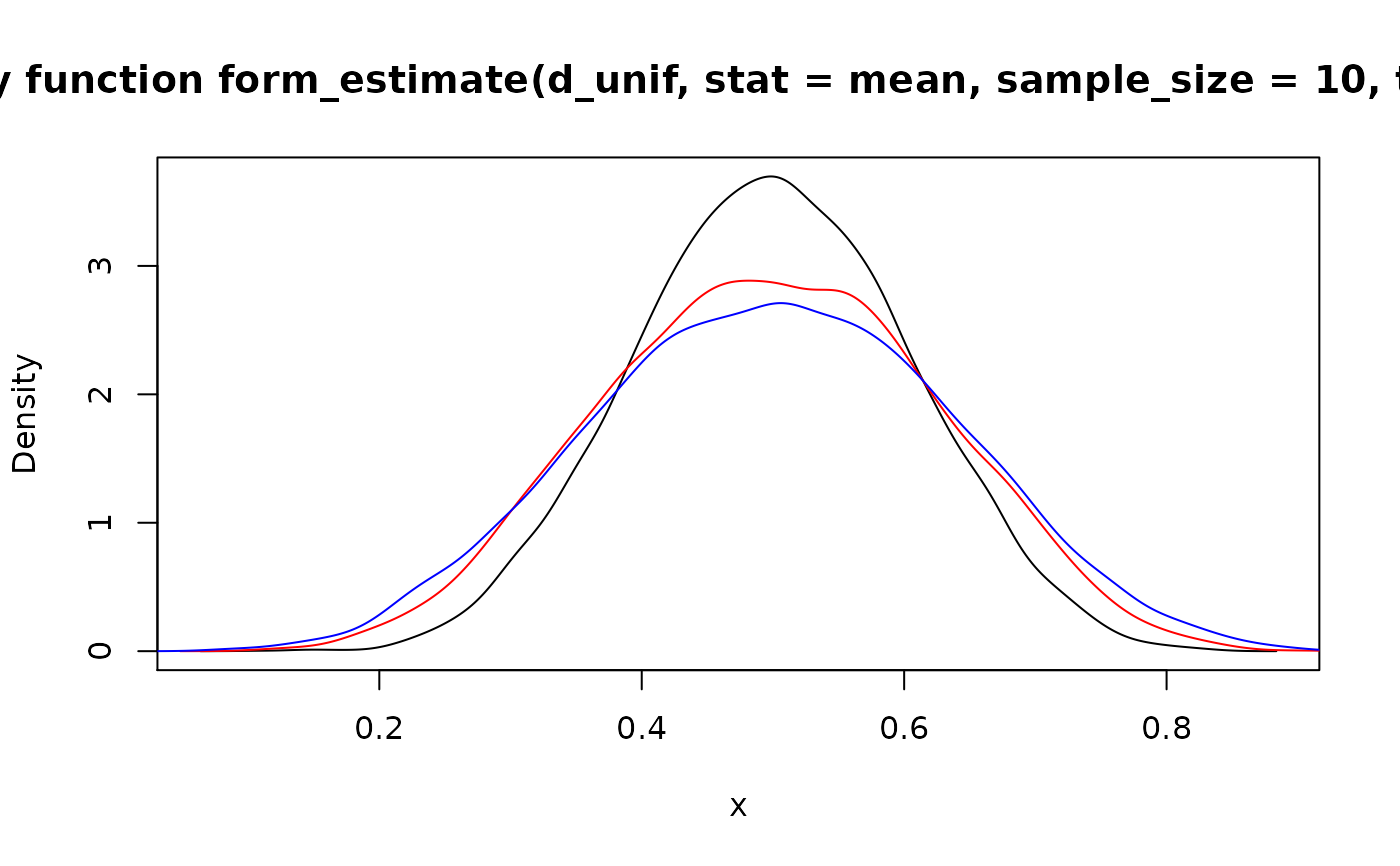Based on pdqr-function, statistic function, and sample size describe the distribution of sample estimate. This might be useful for statistical inference.

form_estimate(f, stat, sample_size, ..., n_sample = 10000, args_new = list())

Arguments

f A pdqr-function. Statistic function. Should be able to accept numeric vector of size sample_size and return single numeric or logical output. Size of sample for which distribution of sample estimate is needed. Other arguments for stat. Number of elements to generate from distribution of sample estimate. List of extra arguments for new_*() function to control density().

Value

A pdqr-function of the same class and type (if not forced otherwise in args_new) as f.

Details

General idea is to create a sample from target distribution by generating n_sample samples of size sample_size and compute for each of them its estimate by calling input stat function. If created sample is logical, boolean pdqr-function (type "discrete" with elements being exactly 0 and 1) is created with probability of being true estimated as share of TRUE values (after removing possible NA). If sample is numeric, it is used as input to new_*() of appropriate class with type equal to type of f (if not forced otherwise in args_new).

Notes:

• This function may be very time consuming for large values of n_sample and sample_size, as total of n_sample*sample_size numbers are generated and stat function is called n_sample times.

• Output distribution might have a bias compared to true distribution of sample estimate. One useful technique for bias correction: compute mean value of estimate using big sample_size (with mean(as_r(f)(sample_size))) and then recenter distribution to actually have that as a mean.

Examples

# These examples take some time to run, so be cautious # \donttest{ set.seed(101) # Type "discrete" d_dis <- new_d(data.frame(x = 1:4, prob = 1:4 / 10), "discrete") ## Estimate of distribution of mean form_estimate(d_dis, stat = mean, sample_size = 10)
#> Probability mass function of discrete type #> Support: [1.8, 4] (23 elements)
## To change type of output, supply it in args_new form_estimate( d_dis, stat = mean, sample_size = 10, args_new = list(type = "continuous") )
#> Density function of continuous type #> Support: ~[1.67226, 4.12774] (511 intervals)
# Type "continuous" d_unif <- as_d(dunif) ## Supply extra named arguments for stat in ... plot(form_estimate(d_unif, stat = mean, sample_size = 10, trim = 0.1))
lines( form_estimate(d_unif, stat = mean, sample_size = 10, trim = 0.3), col = "red" )
lines( form_estimate(d_unif, stat = median, sample_size = 10), col = "blue" )# Statistic can return single logical value d_norm <- as_d(dnorm) all_positive <- function(x) { all(x > 0) } ## Probability of being true should be around 0.5^5 form_estimate(d_norm, stat = all_positive, sample_size = 5)
#> Probability mass function of discrete type #> Support: [0, 1] (2 elements, probability of 1: 0.0318)
# }